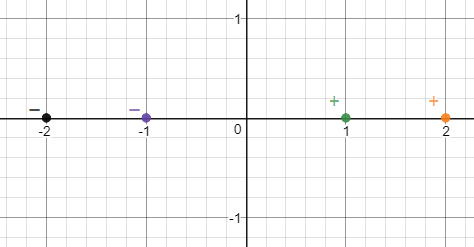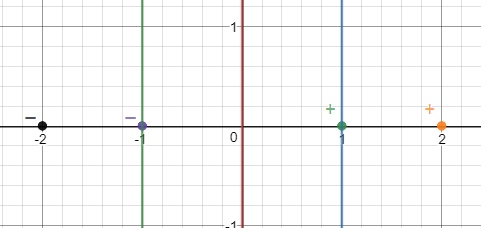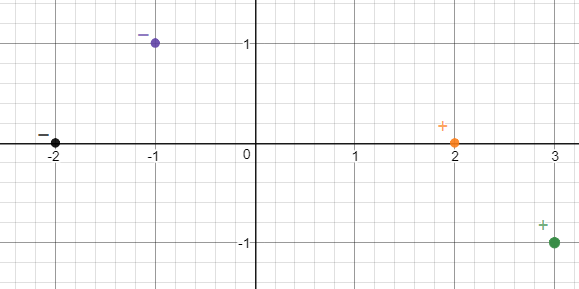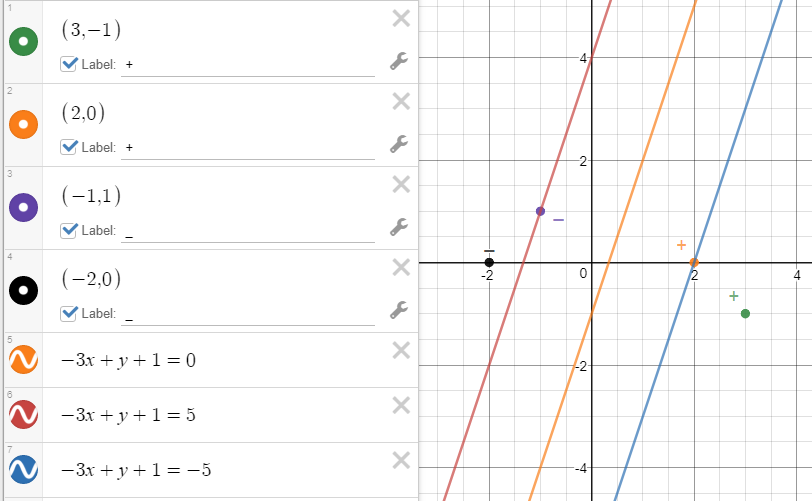# SVM Manual Maximization Procedure

1. Start from idiot example:

4 sample data:  +(1,0), +(2,0), -(-1,0),-(-2,0), what is the SVM boundary and support vectors?It is easy to know that the most closest positive (+) example and negative (-) example are (1,0) and (-1,0) accordingly.  It is easy to know the SVM boundary should be x=0.

Ok, how can we use math analytic to get it via SVM concept, i.e. max the width between closet positive/negative samples.

1. Set the SVM boundary is ax+by+c=0

The support vector are ax+by+c=d and is ax+by+c=-d.   (d>0)

Set the two support vectors distance to the boundary as W

Now, we need to calculate the a,b,c,d to max the width W

1. As (-1,0) and (1,0) are support vectors, according to point to line distance theory (d=|ax0+by0+c)/sqrt(a^2+b^2)),  W=|d|/ sqrt(a^2+b^2)
2. As (-1,0) and (1,0) satisfied support vectors, we got
a*1+b*0+c=d     and   a*(-1)+b*0+c=-d

Solve the equation systems, we got c=0, a=d

1. Let’s see how to max W:

W=|d|/ sqrt(a^2+b^2)= |a|/ sqrt(a^2+b^2)= 1/ sqrt(1+(b/a)^2)

So, to max W, we need to minimize (b/a)^2, so b/a=0

That is said, b=0.

1. Finally, we can write the boundary equation ax+by+c=0 as ax+0*y+0=0

That is x=0

Also, we will get the support vector are ax=a, and ax=-a,

That is x=1 and x=-1.

Below is the diagram for the solved boundary(x=0) and two support vectors(x=1 and x=-1).2) a little bit complex example:1. As (-1,1) and (2,0) are support vectors, according to point to line distance theory (d=|ax0+by0+c)/sqrt(a^2+b^2)),  W=|d|/ sqrt(a^2+b^2)
2. As (-1,1) and (2,0) satisfied support vectors, we got
a*2+b*0+c=d     and   a*(-1)+b*1+c=-d

Solve the equation systems, we got c=(-a-b)/2, d=(3a-b)/2

1. Let’s see how to max W:

W=|d|/ sqrt(a^2+b^2)= |(3a-b)/2|/ sqrt(a^2+b^2)= |(3-b/a)/2|/  sqrt(1+(b/a)^2) = |(3-e)/2|/  sqrt(1+(e)^2)

So, to max W, that is same as max of (3-e)^2/(1+e^2), we can get it via derivative, e=-1/3

That is said, b/a= -1/3,  a=-3b, max W=|(3-e)/2|/  sqrt(1+(e)^2)=sqrt(10)/2

So, c=(-a-b)/2=b, d=(3a-b)/2=-5b

1. Finally, we can write the boundary equation ax+by+c=0 as -3bx+b*y+b=0

That is -3x+y+1=0.  The support vectors are

-3x+y+1=-5;    -3x+y+1=5

Below is the diagram for the solved boundary and two support vectors.If we do not calculate and just use the middle line of -1 and 2, that is x=0.5 as boundary, the width (from support vector to boundary line) will be 1.5, which is less that max value of sqrt(10)/2=1.5811.